# ML Aggarwal Class 8 Solutions for ICSE Maths Chapter 7 Percentage Check Your Progress

## ML Aggarwal Class 8 Solutions for ICSE Maths Chapter 7 Percentage Check Your Progress

Question 1.
Convert $$\frac{7}{24}$$ into a percentage correct to foursignificant figures.
Solution: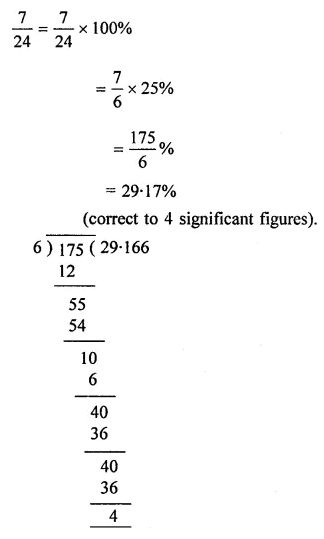Question 2.
Express 750 mL as a percentage of 4 litres.
Solution: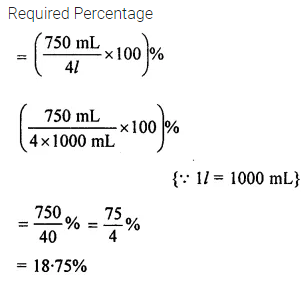Question 3.
A football team won 9 matches out of the total number of matches they played. If their win percentage was 45, then how many matches did they play in all?
Solution: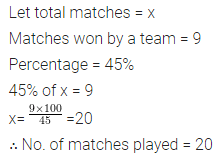Question 4.
In a straight student election, Rahul got 66% of the votes polled. If the defeated student got 187 votes, find the total number of votes polled.
Solution:Question 5.
36 kg of gunpowder contains 27 kg nitre, 5.4 kg charcoal and rest sulphur. Find the percentage content of sulphur in the gunpowder.
Solution: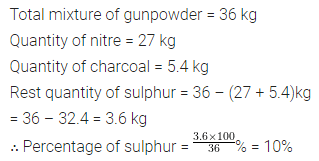Question 6.
75% of the students in a class passed an exam. If 2 more students had passed the exam. 80% would have been successful. How many students are there in the class ?
Solution:Question 7.
The monthly salary of a school teacher in 2007 was ₹12000. It increased by 10% in 2008 and again by 10% in 2009. What is his salary in 2009?
Solution: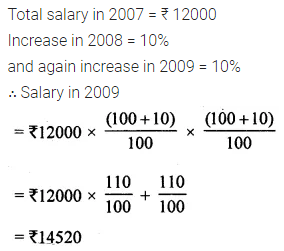Question 8.
Price of a commodity decreased by 10% last year and increased by 20% this year. Find the percentage change in two years.
Solution: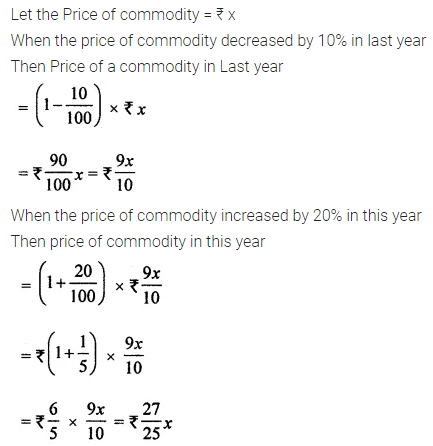Question 9.
A shop gives a 15% discount. What would the sale price of each of these be?
(i) A dress marked at ₹150
(ii) A pair of shoes marked at ₹800.
Solution:Question 10.
A refrigerator marked at ₹18000 is available for ₹17100. Find the discount given and the discount percent.
Solution:Question 11.
Ramu purchased 20 parrots at ₹30 each. Two parrots flew away and Ramu sold the rest of the parrots at ₹40 each. Calculate the profit or loss percentage of Ramu.
Solution:Question 12.
Reena bought two tables for ₹ 1800 each. She sold one at a loss of 8% and the other at a profit of 12%. Find the selling price of each. Also, find out the total profit or loss.
Solution: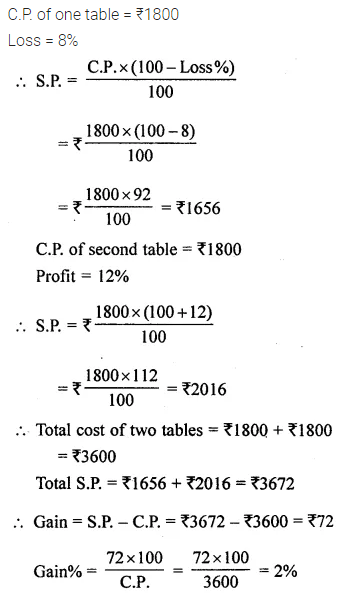Question 13.
Ajit bought an old car ₹78000. He spent ₹2000 on repairs and repainting the car. He sold the car to Anju at a gain of 15%. Anju sold it to Anthony at a loss of 5%. What did the car cost to Anthony?
Solution:Question 14.
Gaurav sold an article at a profit of 12%. Had it been sold for ₹16 more, the profit would have been 20%. Find the C.P. of the article.
Solution: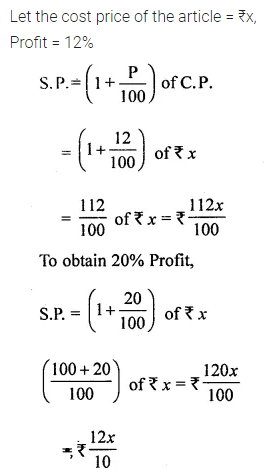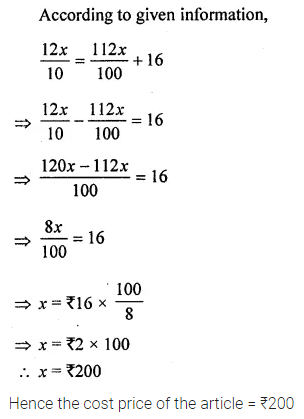Question 15.
A shopkeeper allows 8% discount on his goods and still earns a profit of 15%. If an article is sold for ₹460, find :
(i) the marked price
(ii) the cost price.
Solution: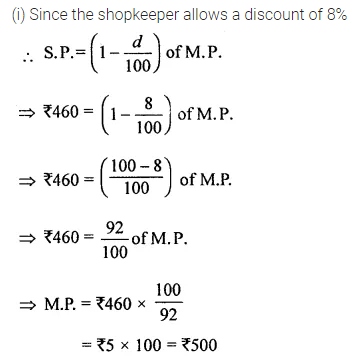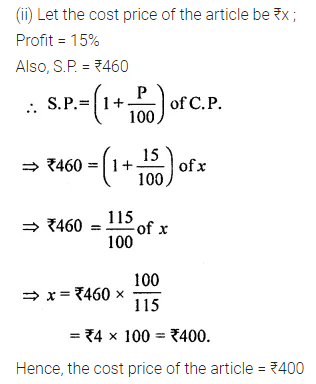Question 16.
Rajesh bought an A.C. for ₹38500 including 10% VAT. Find the price ofA.C. before VAT was added.
Solution:Question 17.
A DVD player is marked for ₹3000. The shopkeeper offers 10% discount on it. If VAT is received 8%. Find the amount paid by the customer.
Solution: